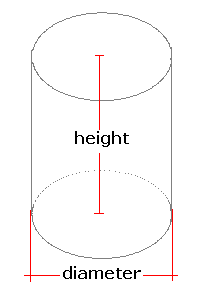Spike's Calculators

# Cylinder Volume and Surface Area

Calculate the volume and surface area of a cylinder.This calculator works for any measurement unit. For example, if you calculate inches, inches will have to be used in all the length fields, and the result of the areas will be square inches; the volume results in cubic inches and length measurements in the starting unit, inches.

### Cylinder

Height of Cylinder #
Diameter #
Decimal Precision #

#### Results:

 Radius # Volume #³ Bottom Area #² Top Area #² Lateral Area #² Surface Area #² Surface to Volume Ratio sa:vol

#### Calculation

• the height of the cylinder
• the diameter of the cylinder
• decimal precision, number of digits after the decimal point

#### Results

• the radius of the cylinder (unit)
• cylinder's volume (unit cubed)
• the base (bottom) area of the cylinder (unit squared)
• the top area of the cylinder (unit squared)
• the lateral area of the cylinder (unit squared)
• the surface area (unit squared)
• surface area to volume ratio (decimal)
##### Formula
```V = ΠD⊃H/4
BA = ΠD²/4;
LS = 2ΠDH/4
SA = 2ΠD²/2+2ΠDH/4
s/v = LS/V
where V is the volume of the cylinder
Π = 3.14159265
D the diameter
H the height
LA, the lateral area of the cone
BA the bottom area of the cylinder
LS is the lateral area of the cylinder
SA the surface area of the cylinder
s/v is the surface area to volume ratio
```